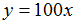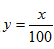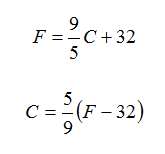Introduction

An inverse function is a function that undoes another function, much like addition undoes subtraction. Inverse functions use inverse operations to turn inputs into outputs and vice versa.

If a function answers the question: “Alice worked this long; how much money has she made?” Then its inverse answers the question: “Alice made this much money; how long did she work?"

If a function answers the question: “How many hours of music fit on 12 CDs?” Then its inverse answers the question: “How many CDs do you need for 3 hours of music?”

If a function is modeled by the rule,then the inverse function is modeled by. Since multiplication and division are inverse operations, they will create inverse functions.

A common example of an inverse function is the Celsius-to-Fahrenheit conversion:where C is the Celsius temperature and F the Fahrenheit temperature.

Substituting the input 100°C into the first function gives an output of 212°F. Inversely, substituting the input 212°F into the second function gives an output of 100°C.